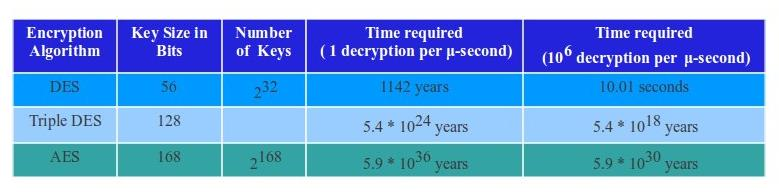NOTIFICATION: These examples are provided for educational purposes. The use of this code and/or information is under your own responsibility and risk. The information and/or code is given ‘as is’. I do not take responsibilities of how they are used.

Before we begin talking about encryption, decryption, and ciphers related topic, let go over some terminologies to have in account:

• Cipher: An algorithm used for encryption.
• Ciphertext: The encrypted(coded) message.
• Cryptanalysis: Study of the principles and methods of deciphering a ciphertext without having the required key.
• Cryptography: Study of the principles and methods of encryption.
• Cryptology: The study of cryptanalysis and cryptography.
• Deciphering: Also known as decryption. The act of transforming a ciphertext to the original plaintext.
• Decryption: Also known as deciphering. The act of transforming a ciphertext to the original plaintext.
• Enciphering: Also known as encryption. The act of transforming a plaintext to a ciphertext.
• Encryption: Also know as enciphering. The act of transforming a plaintext to a ciphertext.
• Plaintext: the original message to be encrypted.
• Product: stages of transposition and substitutions performed.
• Secret key: An input required for the encryption and/or decryption algorithms.
• Substitution: Map each element in a plain text to another element.
• Transposition: Rearrange the elements in the plaintext

Cryptography

A cryptographic system is characterized by the use of encryption operations, number of keys used for encryption and decryption, and the way in which the plain text is processed.

Encryption Operations: In order to encrypt a plaintext to a chipertext is required to perform multiple stages of transposition and substitution, also known as product.

• Substitution: We take each element from the plaintext and mapped them to another element
• Transposition: We  take each element in the plaintext and rearrange its order in such a way that it differ from the original plaintext.

To perform encryption and decryption, we use a key reference. We can categorize the encryption techniques as  symmetric, single, asymmetric, double, and/or public.

The plaintext can be processed by using a method of streams or blocks:

• Stream: The plaintext is processed as a continuous set of elements in which each element is encrypted one at a time.
• Blocks: The plaintext is divided in a set of blocks in which each block is encrypted one at a time.

Cryptanalysis

As explained in the terminology list, Cryptanalysis is purpose of decrypt an encrypted ciphertext without the knowledge of the key used for the encryption. One way is to attack the encryption system and recover the key used for the encryption instead of recovering the plaintext from a single ciphertext.
Cryptanalysis attacks are divided in two categories:

1. Brute-force Attack: Every combination of a possible key is tested on the chipertext until the plaintext is obtained.
2. Cryptanalytic Attack: The use of knowing some characteristic of the original plaintext such as some used keywords, language, format, plaintext to ciphertext pairs examples, and  knowledge of the possible algorithm used to decrypt the ciphertext.

Unconditional Security

We call unconditional security when a cipher cannot be broken by using a ciphertext and the plaintext that produced the ciphertext regardless of the computational power and time available. Up to day, there are no encryption algorithm that can be unconditional secure with the exception of the one-time pad encryption algorithm <http://www.ibm.com/developerworks/library/s-pads.html> which will be explained in the following postings.

Computational Security

Base on the cost-benefit of braking a cipher, a cipher may not be broker due:

1. The cost of braking the cipher is greater than the value of the plaintext encrypted
2. The time required to breaking the cipher exceed the usefulness lifetime of the plaintext encrypted
3. Depending of the complexity of the cipher, there would be a limitation of computing resources and time.

Brute Force Search

As explained before, we call brute force to try every key combination possible to decrypt the ciphertext into plaintext. Before obtaining success, the attacker must try at least 50 percent of the possible keys; therefore, the probability of success may be proportional to the size of the key.

Lets assume we wish to have to option of using: